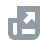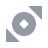フィアット
マーケット
NFT (非代替性トークン)
New
ダウンロード
English
USD
なにかお困りでしょうか?アカウント機能TutorialBinance Fan Tokenバイナンスアーンバイナンス先物取引

USDⓈ-M 先物
COIN-M 先物

ヘッジモード
インデックス
レバレッジトークンファイナンスAPIセキュリティーBinance ConvertNFTVIP
この記事は、現在お客様の言語ではサポートされておりません。英語は自動翻訳を使用して確認することを推奨いたします。

# How to Calculate Liquidation Price of USDⓈ-M Futures Contracts

2020-08-14 02:14
Below is the liquidation price formula for USDⓈ-M futures contracts under the cross margin mode:
where
Notes:
• In Cross margin mode, WB is crossWalletBalance
• In Isolated margin mode, WB is isolatedWalletBalance of the isolated position, TMM=0, UPNL=0, substitute the position quantity, MMR, cum into the formula to calculate.
• Under the cross margin mode, the same ticker/symbol, both long and short position share the same liquidation price except in the isolated mode. Under the isolated mode, each isolated position will have different liquidation prices depending on the margin allocated to the positions.
• If the liquidation price is less than 0, the UI display would be “--”

## Maintenance Margin Rate

You can find the “Maintenance Margin Rate” from the table below with the position value in USDT.
For example, if the position size of a USDT-margined BTCUSDT contract is 264,000 USDT, then the maintenance margin rate would be 1% (or 0.01).
Note:
If your position (calculated at the liquidation price) and the current position (calculated at the opening price) are of different levels, then you must substitute (i.e. to calculate at the liquidation price) the maintenance margin rate and the maintenance margin amount of the position level, to recalculate the liquidation price.

## Maintenance Amount

You can find the “Maintenance Amount” from the table below with the position value in USDT. For example, if the position size of a USDT-margined BTC/USDT Contract is 500,000 USDT, then the maintenance amount would be 1,300 USDT.

## Maintenance Amount formula

= [ Floor of Position Bracket on Level n * difference between Maintenance Margin Rate on Level n and Maintenance Margin Rate on Level n-1) ] + Maintenance Amount on Level n-1
For example, the Maintenance Amount on Level 3
= 250,000 * (1% - 0.5%) + 50 USDT
= 1,300 USDT
75x USDT-Margined Perpetual Contract:
* ADAUSDT, BNBUSDT, DOTUSDT, EOSUSDT, ETCUSDT, LINKUSDT, LTCUSDT, TRXUSDT, XLMUSDT, XMRUSDT, XRPUSDT, XTZUSDT, BCHUSDT

## Example: Calculating Liquidation Price in One-way & Cross Margin mode

(WB) Wallet Balance = 1,535,443.01
You may find your maintenance margin rates and amounts through the tables above, as highlighted in the following image.
For ETHUSDT:
Taking into account that Maintenance margin = Notional value * Maintenance Margin rate-cum
Notional Value = Price * Size
Maintenance Margin of ETHUSDT = 356,512.508 (4918775.081 * 10.00% - 135365).
(WB) Wallet Balance = 1,535,443.01
(TMM1) Maintenance Margin of all other contracts, excluding Contract 1 = 71200.81144
(UPNL1) Unrealized PNL of all other contracts, excluding Contract 1 = -56,354.57
(cumB) Maintenance Amount of BOTH position (one-way mode) = 135,365.00
(cumL) Maintenance amount of LONG position (hedge mode) = 0
(cumS) Maintenance amount of SHORT position (hedge mode) = 0
(Side1BOTH) Direction of BOTH position, 1 as long position, -1 as short position = 1
(Position1BOTH) Absolute value of BOTH position size (one-way mode) = 3,683.979
(EP1BOTH) Entry Price of BOTH position (one-way mode) =1,456.84
(Position1LONG) Absolute value of LONG position size (hedge mode) = 0
(EP1LONG) Entry Price of LONG position (hedge mode) = 0
(Position1SHORT) Absolute value of SHORT position size (hedge mode) = 0
(EP1SHORT) Entry Price of SHORT position (hedge mode) = 0
(MMRB) Maintenance margin rate of BOTH position (one-way mode) = 10%
(MMRL) Maintenance margin rate of LONG position (hedge mode) = 0
(MMRS) Maintenance margin rate of SHORT position (hedge mode) = 0

### For BTCUSDT:

Taking into account that Maintenance margin=Notional value*Maintenance Margin rate-cum
Notional Value = Price * Size
Maintenance Margin of BTCUSDT = 71200.81144 (3500032.458 * 2.50%% - 16300).
(WB) Wallet Balance = 1,535,443.01
(TMM1) Maintenance Margin of all other contracts, excluding Contract 1 = 356,512.508
(UPNL1) Unrealized PNL of all other contracts, excluding Contract 1 = -448,192.89
(cumB) Maintenance Amount of BOTH position (one-way mode) = 16,300.000
(cumL) Maintenance amount of LONG position (hedge mode) = 0.
(cumS) Maintenance amount of SHORT position (hedge mode) = 0
(Side1BOTH) Direction of BOTH position, 1 as long position, -1 as short position = 1
(Position1BOTH) Absolute value of BOTH position size (one-way mode) = 109.488
(EP1BOTH) Entry Price of BOTH position (one-way mode) =32,481.980
(Position1LONG) Absolute value of LONG position size (hedge mode) = 0
(EP1LONG) Entry Price of LONG position (hedge mode) = 0
(Position1SHORT) Absolute value of SHORT position size (hedge mode) = 0
(EP1SHORT) Entry Price of SHORT position (hedge mode) = 0
(MMRB) Maintenance margin rate of BOTH position (one-way mode) = 2.50%
(MMRL) Maintenance margin rate of LONG position (hedge mode) = 0
(MMRS) Maintenance margin rate of SHORT position (hedge mode) = 0

### Liquidation Price Formula:

With this information and by inputting the appropriate information in the formula we can see that the Liquidation Price for the ETHUSDT contract = 1153.26 and for BTCUSDT =26,316.89
Please note that there may be a slight variation due to decimals.